# Irrigatable Area

This calculator finds the land area that can be irrigated with a given flow of water. The minimum system capacity (supply) is the available water from the supply. The water needs is the peak crop water need during a specific time period. The available hours of operation per day is measured as the available hours for irrigation on a worst case day. The system efficiency is based on the irrigation efficiency and distribution uniformity.

## Irrigatable Area As Limited By Water Supply

Minimum System Capacity (Supply):
Water Needs:
Operation Hours Per Day:
System Efficiency:
Irrigated Area:

### The Equation

This calulator uses this formula to determine the Irrigatable area.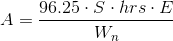Where: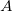= Irrigatable Area (sq. ft)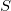= Minimum system capacity, or supply (gpm)= Water needs (in/day)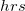= Operation hours per day (hrs)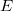= System Efficiency (as a decimal)

Reference: Washington State University